# A loop is moved toward a current-carrying wire as shown below. As the wire is moving,...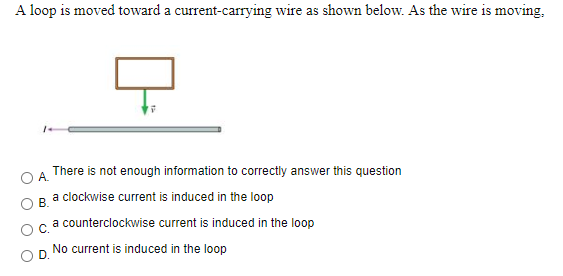A loop is moved toward a current-carrying wire as shown below. As the wire is moving, A B There is not enough information to correctly answer this question a clockwise current is induced in the loop a counterclockwise current is induced in the loop No current is induced in the loop D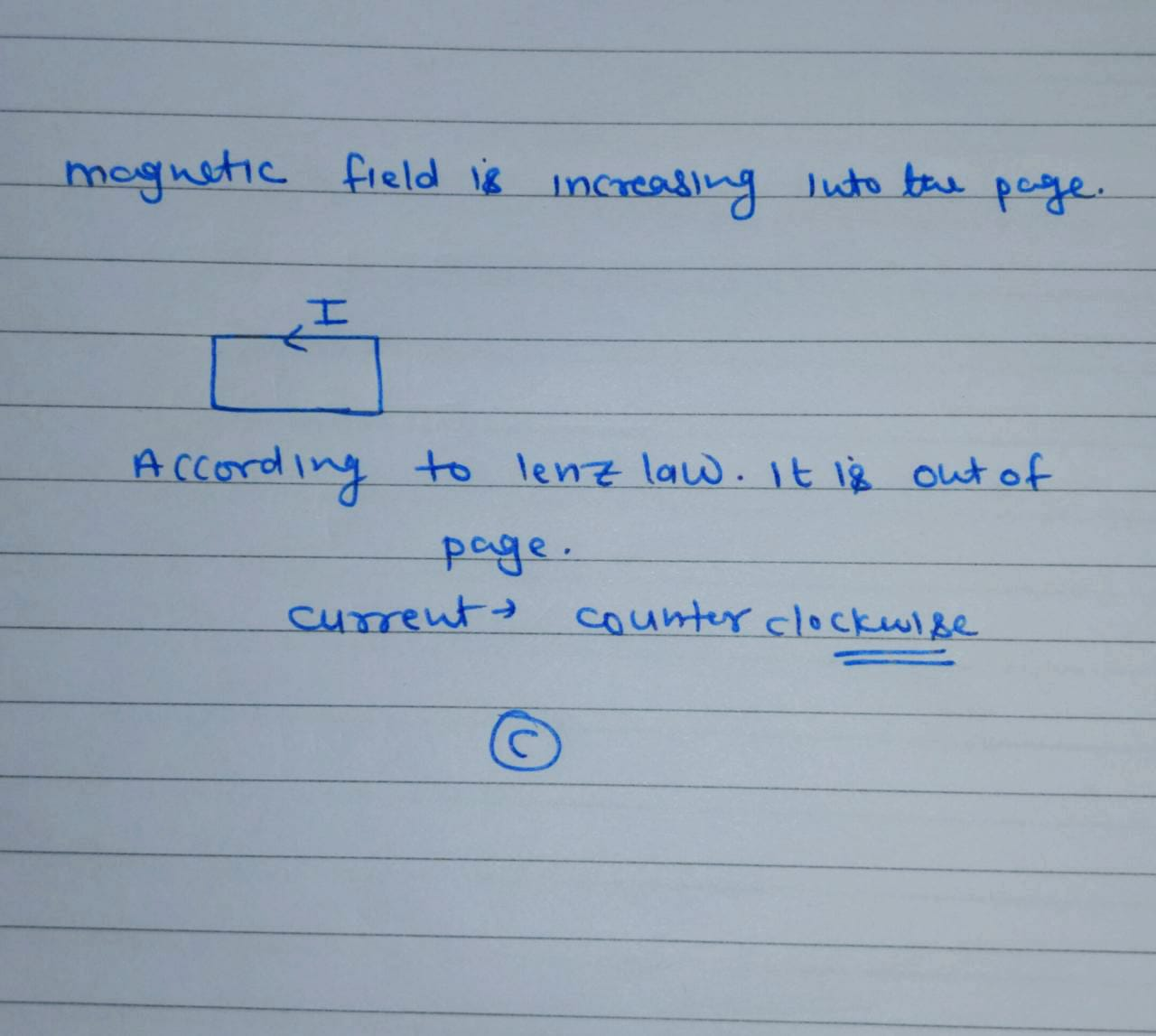##### Add Answer of: A loop is moved toward a current-carrying wire as shown below. As the wire is moving,...
Similar Homework Help Questions
• ### Please explain why the answer is "Counterclockwise." A circular conducting loop is being moved toward a current-carrying wire (upward in the figure) at a constant speed. What will be the dire...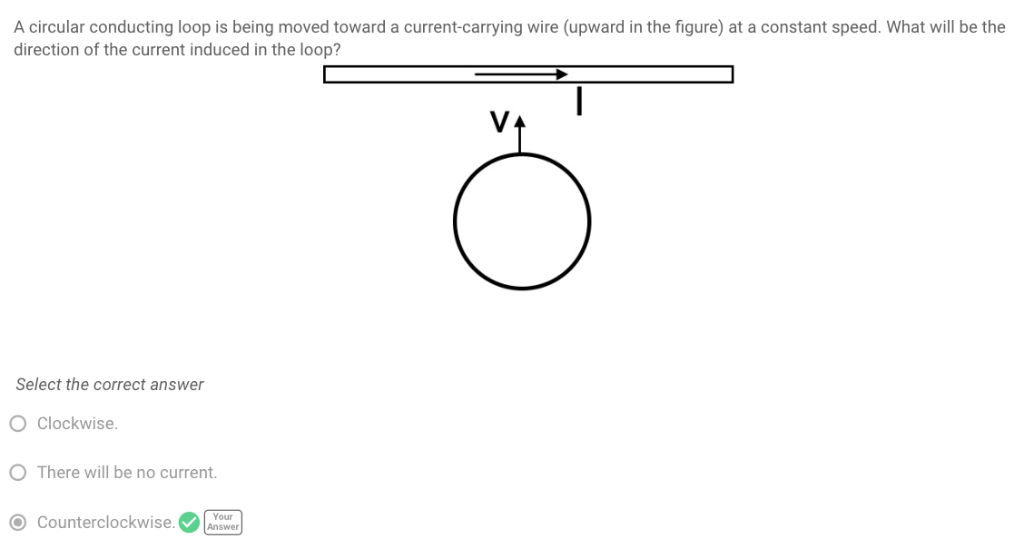Please explain why the answer is "Counterclockwise." A circular conducting loop is being moved toward a current-carrying wire (upward in the figure) at a constant speed. What will be the direction of the current induced in the loop? Select the correct answer O Clockwise There will be no current Counterclockwisenwer A circular conducting loop is being moved toward a current-carrying wire (upward in the figure) at a constant speed. What will be the direction of the current induced in the...

• ### 4) (12 pts) A long straight wire carrying a current I, is near a wire loop...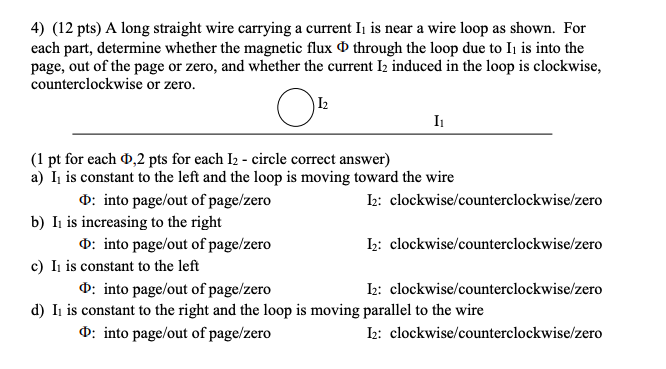4) (12 pts) A long straight wire carrying a current I, is near a wire loop as shown. For each part, determine whether the magnetic flux 0 through the loop due to Ii is into the page, out of the page or zero, and whether the current 12 induced in the loop is clockwise, counterclockwise or zero. 12 I O (1 pt for each 0,2 pts for each 12 - circle correct answer) a) I, is constant to the left...

• ### (5 Points) The figure below shows a rectangular loop of wire moving away from a long...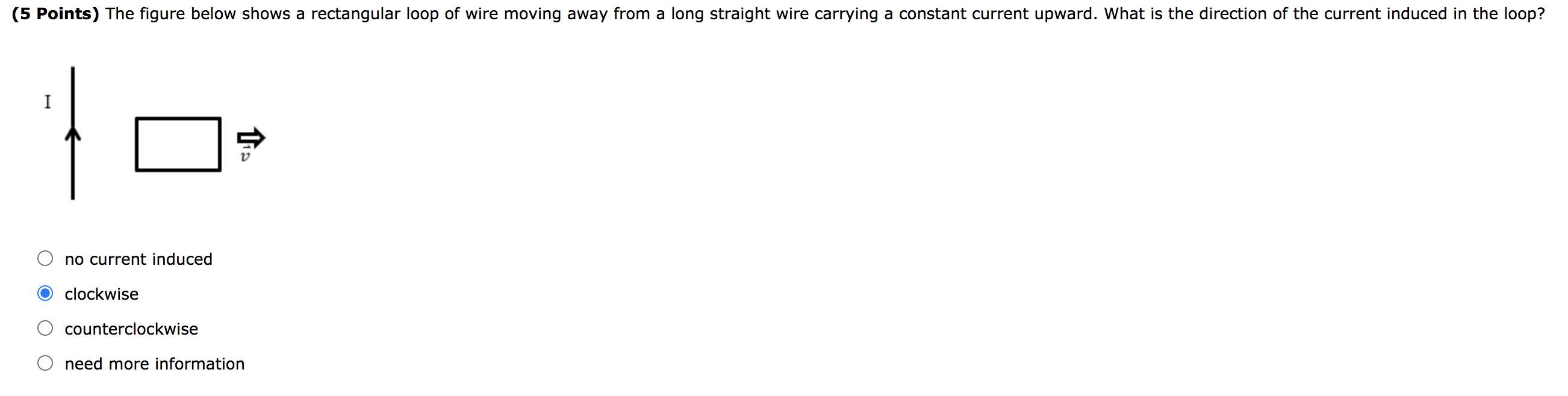(5 Points) The figure below shows a rectangular loop of wire moving away from a long straight wire carrying a constant current upward. What is the direction of the current induced in the loop? I This no current induced clockwise counterclockwise d need more information

• ### The current I in a long, straight wire is constant and is directed toward the right...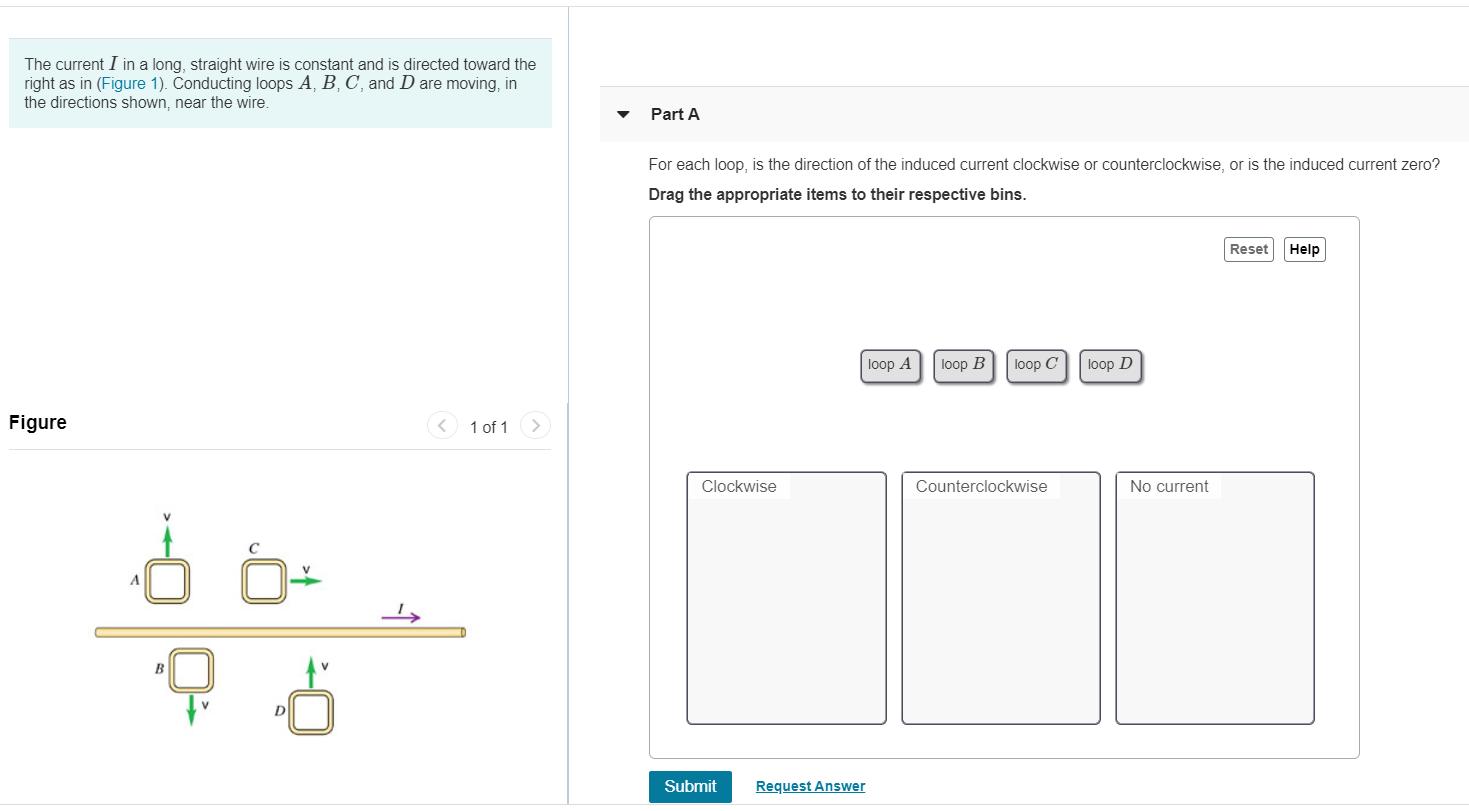The current I in a long, straight wire is constant and is directed toward the right as in (Figure 1). Conducting loops A, B, C, and D are moving, in the directions shown, near the wire. Part A For each loop, is the direction of the induced current clockwise or counterclockwise, or is the induced current zero? Drag the appropriate items to their respective bins. Reset Help loop A loop B loop C loop D Figure 1 of 1 >...

• ### (5 Points) The figure below shows a rectangular loop of wire moving away from a long...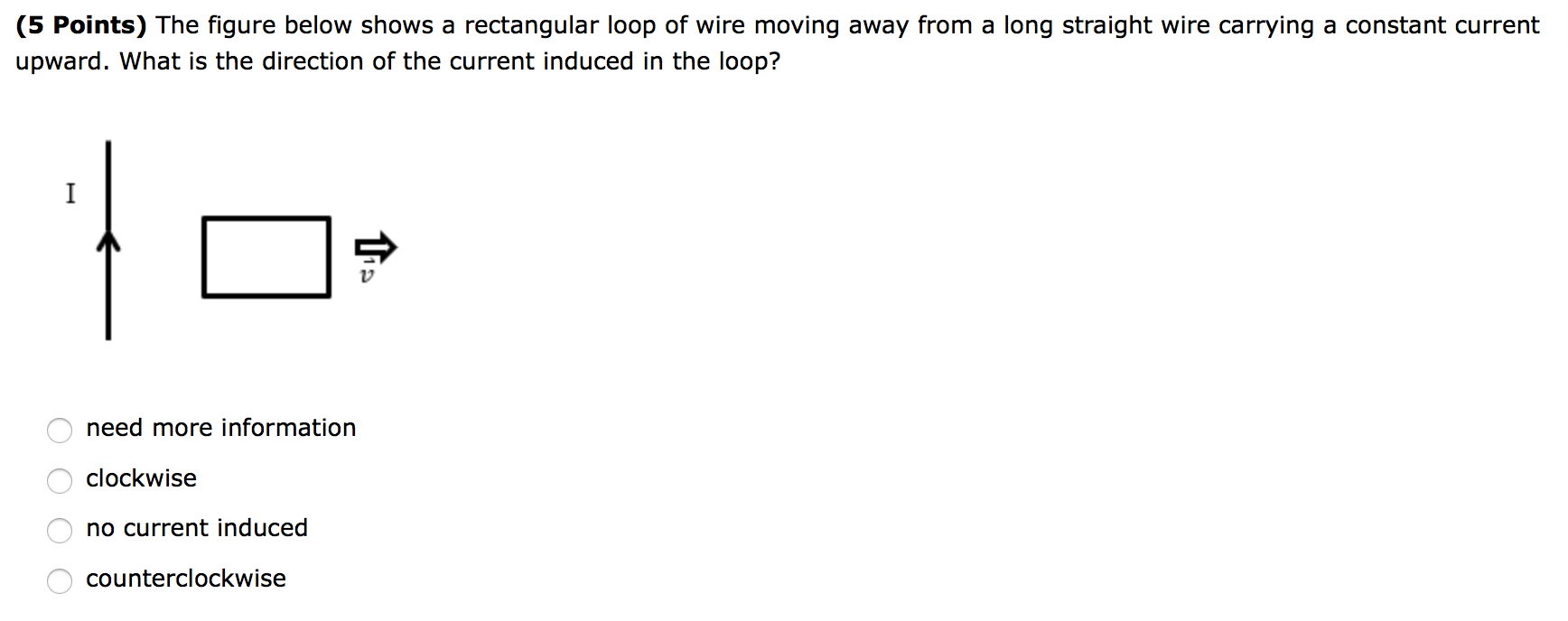(5 Points) The figure below shows a rectangular loop of wire moving away from a long straight wire carrying a constant current upward. What is the direction of the current induced in the loop? I need more information clockwise ОООО no current induced counterclockwise

• ### Q4. (5 points) A wire carries a constant current 1. If a loop is moving toward...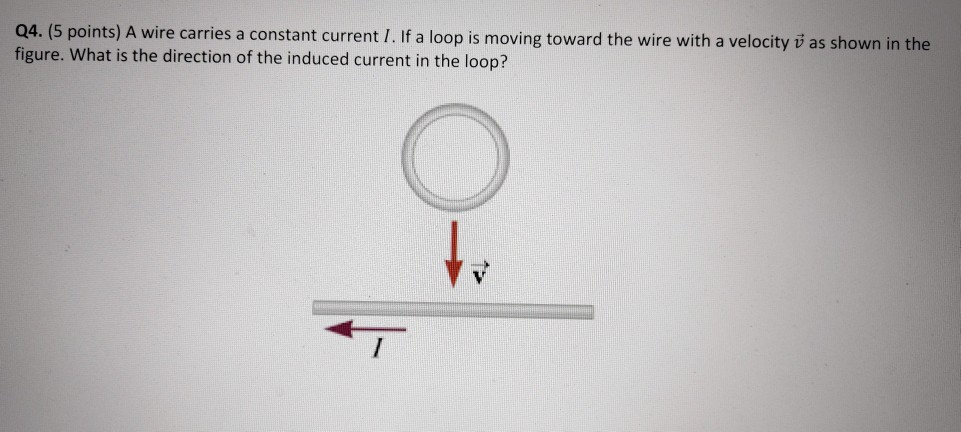Q4. (5 points) A wire carries a constant current 1. If a loop is moving toward the wire with a velocity v as shown in the figure. What is the direction of the induced current in the loop? to

• ### Conducting Loop and Current-Carrying WireAn infinite straight wire carries current I1 = 5.8 A in the positive y-direction as shown. At time t = 0, a conducting wire, aligned with the y-direction islocated a distance d = 51 cm from the y-axis and moves with velocity v = 19 cm/s in the negaitve x-direction as shown. The wire has length W = 24 cm.1) What is ε(0), the emf induced in the moving wire at t = 0? Define the emf to be positive...

• ### conducting loops and current carrying wire1. What is ε(0), the emf induced in the moving wire at t = 0? Define the emf to be positive if the potential at point a is higher than that at point b.2. What is ε(t1), the emf induced in the moving wire at t = t1 = 2.9 s? Define the emf to be positive if the potential at point ais higher than that at point b.3. The wire is now replaced by a conducting rectangular loop as...

• ### The figure shows a stationary conducting loop adjacent to a horizontal current-carrying wire. For which of...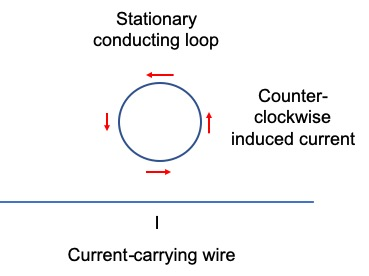The figure shows a stationary conducting loop adjacent to a horizontal current-carrying wire. For which of the following conditions will a counter-clockwise induced current circulate in the stationary loop? a) The current in the horizontal wire flows to the right and its value is increasing. b) The current in the horizontal wire flows to the right and its value is constant. c) The current in the horizontal wire flows to the left and its value is decreasing. d) The current...

• ### The figure shows a stationary conducting loop adjacent to a horizontal current-carrying wire. For which of...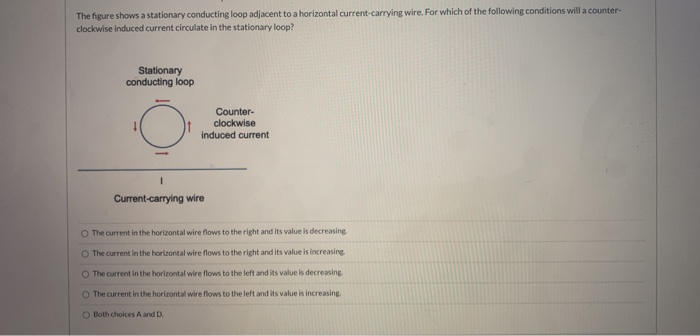The figure shows a stationary conducting loop adjacent to a horizontal current-carrying wire. For which of the following conditions will a counter- clockwise induced current circulate in the stationary loop? Stationary conducting loop Counter- clockwise induced current Current-carrying wire The current in the horizontal wire flows to the right and its value is decreasing The current in the horizontal wire flows to the right and its value is increasing The current in the horizontal wire flows to the left and...

Free Homework App